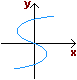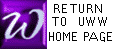WHICH GRAPHS ARE FUNCTIONS?

 THE VERTICAL LINE TEST A graph (or set of points) in the plane is a FUNCTION if no vertical line contains more than one of its points.

 Is this graph a function?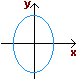Is this graph a function?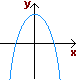Is this graph a function?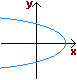Is this graph a function?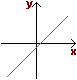Is this graph a function?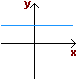Is this graph a function?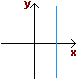Is this graph a function?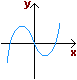Is this graph a function?